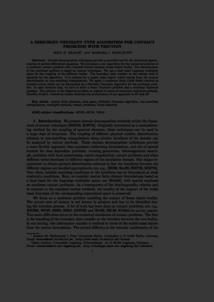Repository: Freie Universität Berlin, Math Department

# A Dirichlet-Neumann type algorithm for contact problems with friction

Krause, R. and Wohlmuth, B. (2002) A Dirichlet-Neumann type algorithm for contact problems with friction. Computing and Visualization in Science, 5 (3). pp. 139-148. ISSN 1432-9360Preview

585kB

Official URL: https://doi.org/10.1007/s00791-002-0096-2

## Abstract

Domain decomposition techniques provide a powerful tool for the numerical approximation of partial differential equations. We introduce a new algorithm for the numerical solution of a nonlinear contact problem with Coulomb friction between linear elastic bodies. The discretization of the nonlinear problem is based on mortar techniques. We use a dual basis Lagrange multiplier space for the coupling of the different bodies. The boundary data transfer at the contact zone is essential for the algorithm. It is realized by a scaled mass matrix which results from the mortar discretization on non-matching triangulations. We apply a nonlinear block Gauß-Seidel method as iterative solver which can be interpreted as a Dirichlet-Neumann algorithm for the nonlinear problem. In each iteration step, we have to solve a linear Neumann problem and a nonlinear Signorini problem. The solution of the Signorini problem is realized in terms of monotone multigrid methods. Numerical results illustrate the performance of our approach in 2D and 3D.

Item Type: Article Mathematical and Computer Sciences > Mathematics > Numerical Analysis Department of Mathematics and Computer Science > Institute of Mathematics 1837 Ekaterina Engel 06 Mar 2016 12:34 03 Mar 2017 14:42

Repository Staff Only: item control page